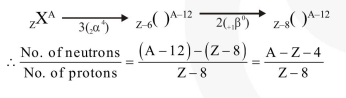# A radioactive nucleus initial mass number A and atomic number Z`
Question:

A radioactive nucleus (initial mass number $A$ and atomic number $Z$ ) emits $3 \alpha$-particles and 2 positrons. The ratio of number of neutrons to that of protons in the final nucleus will be:-

1. $\frac{\mathrm{A}-\mathrm{Z}-4}{\mathrm{Z}-2}$

2. $\frac{A-Z-8}{Z-4}$

3. $\frac{\mathrm{A}-\mathrm{Z}-4}{\mathrm{Z}-8}$

4. $\frac{\mathrm{A}-\mathrm{Z}-12}{\mathrm{Z}-4}$

Correct Option: , 3

Solution: